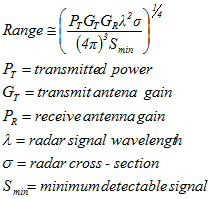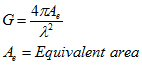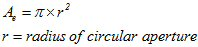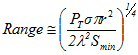New for March 2019. Let's start by looking at some excellent videos from Engineering Funda.  That YouTube channel has over 60,000 subscribers, more than 7M views of hundreds of videos.  Maybe this is the future of education!  But there will always be a place for written content, so that engineers can search and scroll for what they are looking for.  Like Microwaves101...

In the next video, the speaker analyses factors affecting radar range  If you work in radar, you need to memorize these relationships.

Factor affecting radar range by Engineering Funda

Here is Microwaves101 "organic" content on the range equation. Don't you love that word organic?  It often refers to stuff that should be on a compost pile...

But wait, there are exceptions to the radar range equation! One factor that is not covered in the "simple" radar range equation is atmospheric attenuation.  Learn about that on this page.  Later, we'll add this factor to the equations.

Below is the equation for range in a two-way (round-trip) monostatic radar:(1)

The radar range equation can take many forms, in terms of energy, antenna diameter, receive noise figure, etc. And the "range" will be different in a bi-static radar.

Let's examine the range equation from the physical size of an aperture that is shared by transmit and receive. The gain of an antenna is:(2)

For a circular aperture the area is:(3)

Substituting (2) and (3) into (1) we get:(4)

From the equation it is easy to see that in order to double range, you must increase power by 16 times (12 dB!) if everything else is kept constant. Or you could quadruple the frequency (one fourth the wavelength), or double the radius of the aperture. In radar range, aperture area is the most important thing to consider! Note that if you increase the frequency or antenna area, the change in gain means it takes more beams to search a given volume.

Author : Unknown Editor